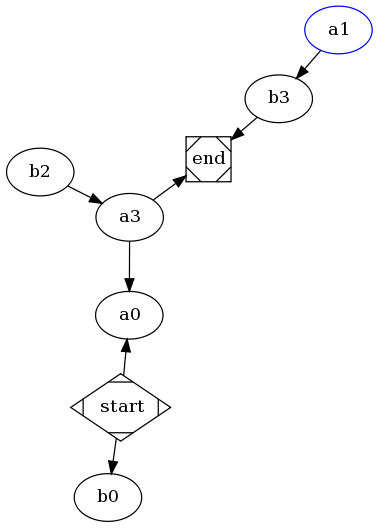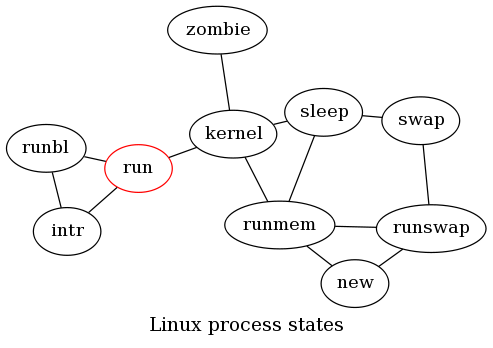# Drawing graphs with graphviz

Graphviz is a great resource for drawing both directed and undirected graphs.

DOT draws directed graphs as hierarchies.

NEATO is a program that makes layouts of undirected graphs.

Here are the two graph examples:

Example 1:

``````digraph G1 {
start -> a0;
start -> b0;
a1 -> b3;
b2 -> a3;
a3 -> a0;
a3 -> end;
b3 -> end;
start[shape=Mdiamond];
end[shape=Msquare];
a1[color=blue;];
}
``````Example 2:

``````graph G2 {
run[color=red];
run -- intr ;
intr -- runbl;
runbl -- run;
run -- kernel;
kernel -- zombie;
kernel -- sleep;
kernel -- runmem;
sleep -- swap;
swap -- runswap ;
runswap -- new;
runswap -- runmem ;
new -- runmem;
sleep -- runmem;
overlap=false
label="Linux process states"
fontsize=15;
}
``````To generate the svg:

``````neato -Tsvg   g2.txt -o g2.svg
``````

tags: & category: -